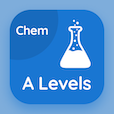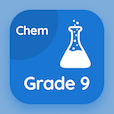Online A Level Courses

A Level Chemistry Practice Tests

A Level Chemistry Tests

The Book Balancing Equations Reactions with oxygen Multiple Choice Questions (MCQ Quiz) PDF, Balancing Equations Reactions with oxygen MCQs download to study online a level chemistry courses. Practice Periodicity Multiple Choice Questions and Answers (MCQs), Balancing Equations Reactions with oxygen quiz answers PDF for ACT test. The eBook Balancing Equations Reactions with oxygen MCQ App Download: electronegativity of period 3 oxides, oxides of period 3 elements, chemistry: oxides, chlorides of period 3 elements test prep for SAT practice test.

The MCQ: Consider the reaction: Na(s) + O2(g) → Na2O(s). Moles of sodium needed to balance the equation would be PDF, Balancing Equations: Reactions with oxygen App Download (Free) with 1, 2, 3, and 4 choices for ACT test. Study balancing equations reactions with oxygen quiz questions, download Google eBook (Free Sample) for SAT test.

## Chemistry: Balancing Equations Reactions with oxygen MCQs

MCQ: Consider the reaction: Na(s) + O2(g) → Na2O(s). Moles of sodium needed to balance the equation would be

A) 1
B) 2
C) 3
D) 4

MCQ: Consider the reaction: P(s) + O2(g) → P4O10(s). Moles of O2(g) needed to balance the equation will be

A) 1
B) 3
C) 5
D) 7

MCQ: Consider the reaction: S(s) + O2(g) → SO2. The state of SO2 in this reaction is

A) liquid
B) solid
C) gaseous
D) semi solid

MCQ: Consider the reaction: Na(s) + O2(g) → Na2O. Moles of oxygen needed to balance the equation are

A) 1
B) 2
C) 3
D) 4

MCQ: Consider the reaction: Al(s) + O2 (g) → Al2O3. Moles of Al(s) needed to balance the equation are

A) 1
B) 2
C) 3
D) 4

### Practice Tests: A Level Chemistry Exam Prep

Download A level Chemistry Quiz App, 9th Grade Chemistry MCQ App, and College Chemistry MCQs App to install for Android & iOS devices. These Apps include complete analytics of real time attempts with interactive assessments. Download Play Store & App Store Apps & Enjoy 100% functionality with subscriptions!